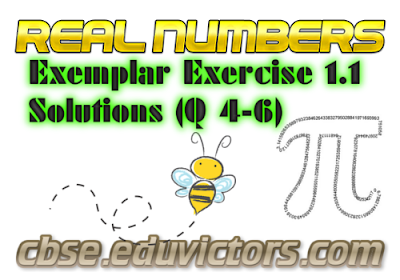## Real Numbers  (NCERT Exemplar Solutions Ex 1.1)

Question 4:
If the HCF of 65 and 117 is expressible in the form 65m − 117, then the value of m is
(A) 4
(B) 2
(C) 1
(D) 3

Answer: (B) 2

Explanation:
Using Euclid’s division algorithm,
b = aq + r, 0 ≤ r < a [∵ dividend = divisor × quotient + remainder]
⇒ 117 = 65 × 1 + 52
⇒ 65 = 52 × 1 + 13
⇒ 52 = 13 × 4 + 0
∴ HCF (65, 117) = 13 .... (i)

Also, given that, HCF (65, 117) = 65m – 117 ... (ii)

From Equations (i) and (ii), we get
65m − 117 = 13
⇒ 65m = 130
⇒ m = 2

Question 5:
The largest number which divides 70 and 125, leaving remainders 5 and 8 respectively, is
(A) 13
(B) 65
(C) 875
(D) 1750

Answer: (A) 13

Explanation:
Since, 5 and 8 are the remainders of 70 and 125, respectively.
Thus, after subtracting these remainders from the numbers, we have the numbers
65  = (70 – 5),
117 = (125 − 8), which is divisible by the required number.
Now, required number = HCF of 65, 117  [Since we need the largest number]
For this, 117 = 65 × 1 + 52 [∵ dividend = divisor × quotient + remainder]
⇒ 65 = 52 × 1 + 13
⇒ 52 = 13 × 4 + 0
∴ HCF = 13
Hence, 13 is the largest number which divides 70 and 125, leaving remainders 5 and 8.

Question 6:
If two positive integers a and b are written and a = x³y² and b = xy³, where x, y are the prime numbers, then HCF (a, b) is
(A) xy
(B) xy²
(C) x³y³
(D) x²y²

Answer: (B) xy²

Explanation:

Given that,
a = x³y² = x × x × x × y × y
b = xy³ = x × y × y × y
∴ HCF of a and b
= HCF (x³y² , xy³)
= x × y × y = xy²
[Since, HCF is the product of the smallest power of each common prime factor involved in the numbers]#### Post a Comment

We love to hear your thoughts about this post!